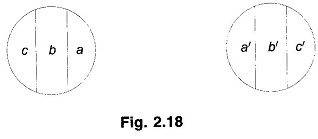## What is Skin Effect and Proximity Effect?:

What is Skin Effect and Proximity Effect? – The distribution of current throughout the cross-section of a conductor is uniform only when DC is passing through it. On the contrary when AC is flowing through a conductor, the current is non-uniformly distributed over the cross-section in a manner that the current density is higher at the surface of the conductor compared to the current density at its centre. This effect becomes more pronounced as frequency is increased. This phenomenon is called skin effect.

It causes larger power loss for a given rms AC than the loss when the same value of DC is flowing through the conductor. Consequently, the effective conductor resistance is more for AC then for DC. A qualitative explanation of the phenomenon is as follows.

Imagine a solid round conductor (a round shape is considered for convenience only) to be composed of annular filaments of equal cross-sectional area. The flux linking the filaments progressively decreases as we move towards the outer filaments for the simple reason that the flux inside a filament does not link it. The inductive reactance of the imaginary filaments therefore decreases outwards with the result that the outer filaments conduct more AC than the inner filaments (filaments being parallel). With the increase of frequency the non-uniformity of inductive reactance of the filaments becomes more pronounced, so also the non-uniformity of current distribution. For large solid conductors the skin effect is quite significant even at 50 Hz. The analytical study of skin effect requires the use of Bessel’s functions.

Apart from the skin effect, non-uniformity of current distribution is also caused by proximity effect. Consider a two-wire line as shown in Fig. 2.18. Each line conductor can be divided into sections of equal cross-sectional area (say three sections). Pairs aa’, bb’ and cc’ can form three loops in parallel. The flux linking loop aa’ (and therefore its inductance) is the least and it increases somewhat for loops bb’ and cc’. Thus the density of AC flowing through the conductors is highest at the inner edges (aa’) of the conductors and is the least at the outer edges (cc’). This type of non-uniform AC current distribution becomes more pronounced as the distance between conductors is reduced. Like skin effect, the non-uniformity of current distribution caused by proximity effect also increases the effective conductor resistance. For normal spacing of overhead lines, this effect is always of a negligible order. However, for underground cables where conductors are located close to each other, proximity effect causes an appreciable increase in effective conductor resistance.Both Skin Effect and Proximity Effect depend upon conductor size, frequency, distance between conductors and permeability of conductor material.

Scroll to Top